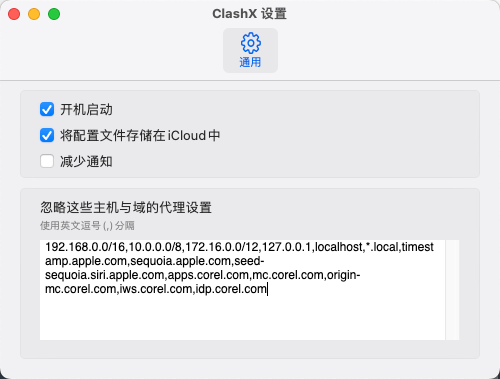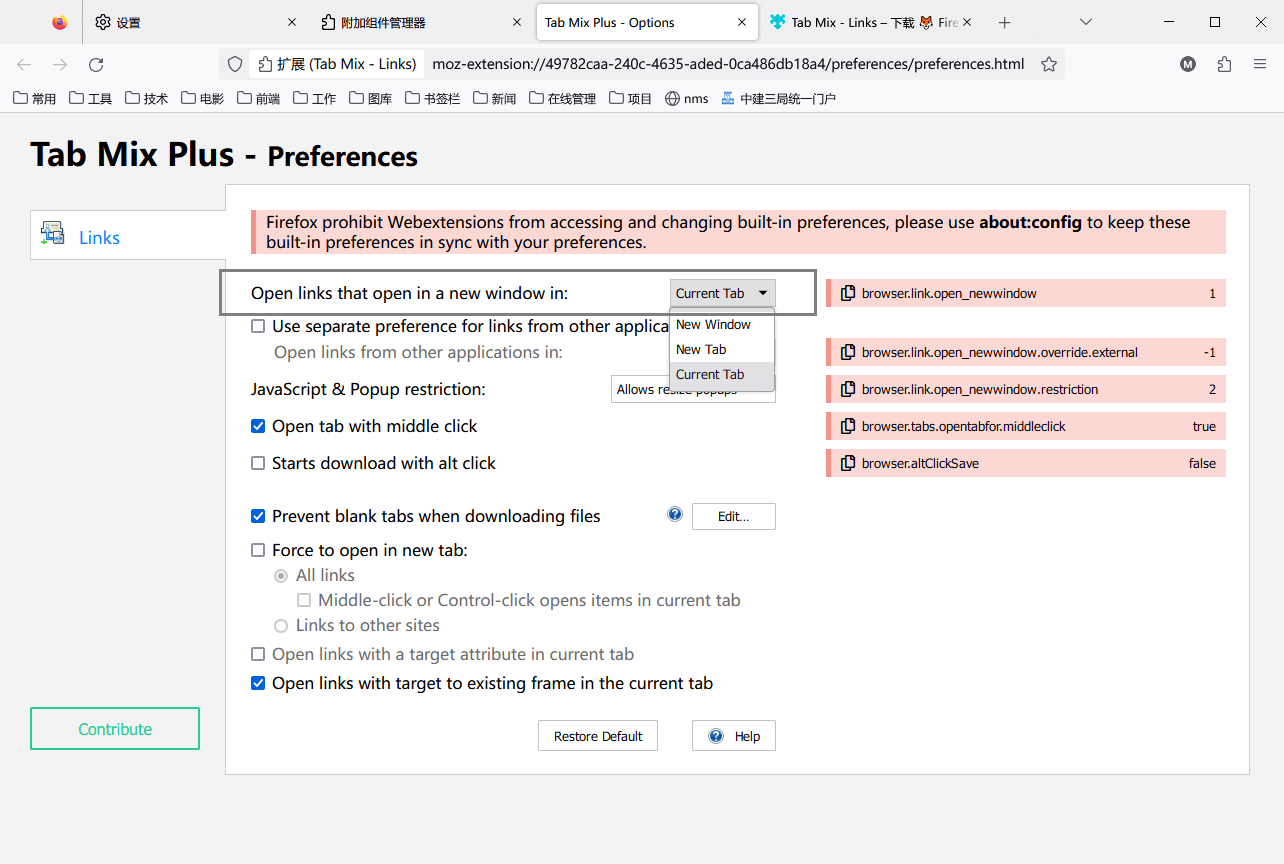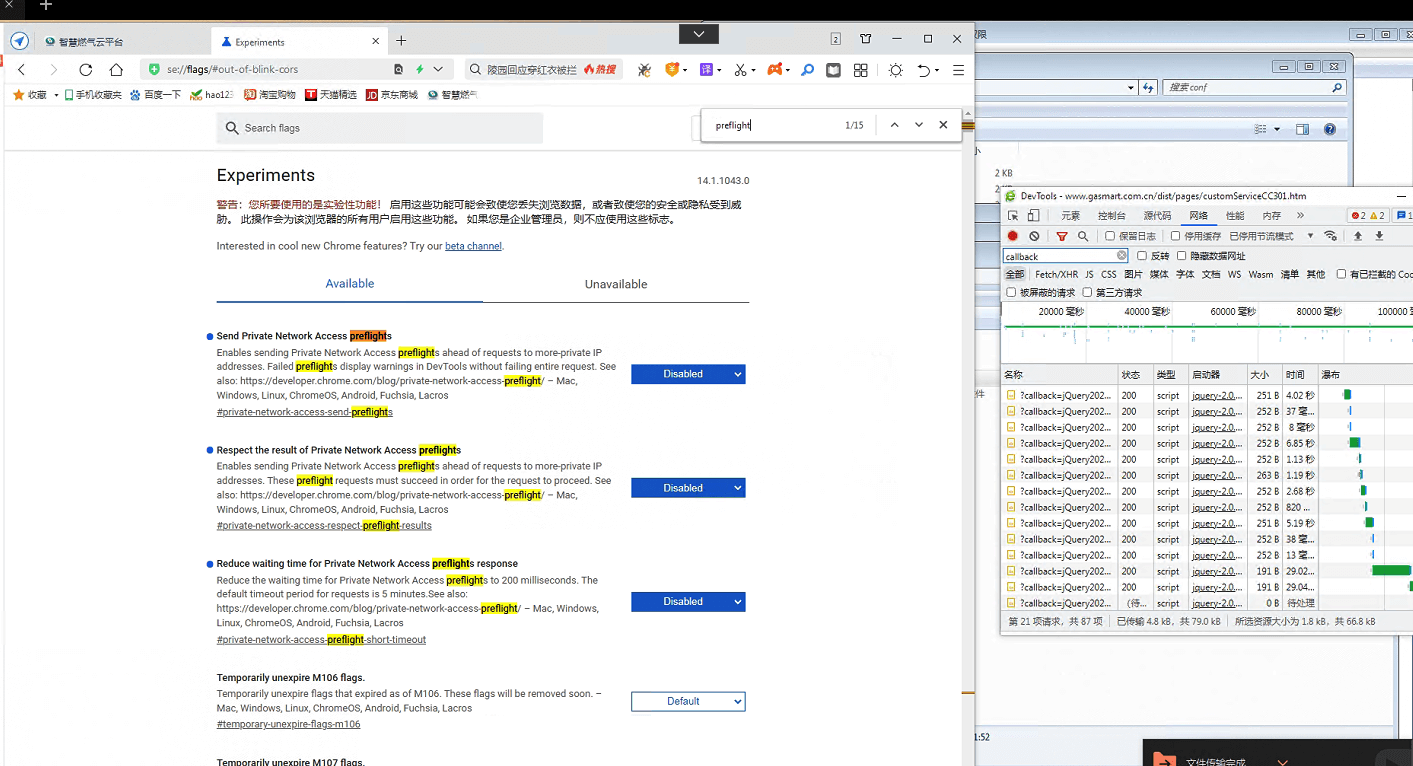## indexOf 与 for 循环性能分析

``````console.clear()
let a = []
// populating array data
for (let i = 0; i < 100000; i++) {
a.push(i)
}
let testNum = 90000
let found
let totalMS4ForLoop = 0
let totalMS4ForIn = 0
let totalMS4IndexOf = 0
let start
let end
// simulating 10000 requests which are come consecutively
for (o = 0; o < 10000; o++) {
start = Date.now()
for (let i = 0; i < a.length; i++) {
if (a[i] == testNum) { found = a[i]; break }
}
end = Date.now()
totalMS4ForLoop += end - start
start = Date.now()
for (let j in a) {
if (a[j] == testNum) { found = a[j]; break }
}
end = Date.now()
totalMS4ForIn += end - start
start = Date.now()
found = a[a.indexOf(testNum)]
end = Date.now()
totalMS4IndexOf += end - start
}
console.log("100000x10000 for-loop executions took total " + totalMS4ForLoop + " ms.")
console.log("100000x10000 for-in executions took total " + totalMS4ForIn + " ms.")
console.log("100000x10000 indexOf executions took total " + totalMS4IndexOf + " ms.")``````

100000x10000 for-loop executions took total 283 ms.
100000x10000 for-in executions took total 33368 ms.
100000x10000 indexOf executions took total 113 ms.

## Java 调用方法 方法名是个变量

``````import java.lang.reflect.Method;

public class Main {
public static void main(String[] args) {
try {
// 创建一个包含方法名的字符串变量
String methodName = "printMessage";

// 获取类Main的Class对象
Class<?> c = Main.class;

// 使用Class对象和方法名获取Method对象
Method method = c.getDeclaredMethod(methodName, String.class);

// 创建一个实例对象
Main mainInstance = new Main();

// 使用Method对象和实例对象调用方法
method.invoke(mainInstance, "Hello, World!");
} catch (Exception e) {
e.printStackTrace();
}
}

public void printMessage(String message) {
System.out.println(message);
}
}``````

## 使用JS，判断是一个变量是否为数字或字符串数字

``````function isNumeric(value) {
// 使用 typeof 来判断变量是否为数字类型
if (typeof value === 'number') {
return true;
}

// 使用正则表达式来检查字符串是否表示数字
if (typeof value === 'string' && value.trim() !== '') {
// 去除字符串两端的空格，并检查是否为空字符串
return /^\d+(\.\d+)?\$/.test(value.trim());
}

return false;
}``````

IDEA端口占用，而实际没有占用

## cdr设置

HOSTS添加

``````127.0.0.1 apps.corel.com
127.0.0.1 mc.corel.com
127.0.0.1 origin-mc.corel.com
127.0.0.1 iws.corel.com
127.0.0.1 idp.corel.com``````

``apps.corel.com,mc.corel.com,origin-mc.corel.com,iws.corel.com,idp.corel.com``## Chrome浏览器的 Ctrl+W 关闭网页的快捷键失效

Chrome浏览器的 Ctrl+W 关闭网页的快捷键经常用着用着不好使了，原因是企业微信的导致的快捷键冲突，关闭企业微信或其他企业微信私有部署的软件，就正常了。记录一下。

## 开启浏览器的单标签模式## js 数组 倒序

``const arr = [1, 2, 3, 4, 5];``

``arr.reverse();``

``````const arr = [1, 2, 3, 4, 5];
const reversedArr = arr.slice().reverse();``````

## js 二维数组 的key 按照指定顺序排序

``````const arr = [
{ name: 'Alice', age: 28 },
{ name: 'Bob', age: 21 },
{ name: 'Charlie', age: 35 },
{ name: 'David', age: 18 }
];``````

``````const order = ['Charlie', 'Bob', 'David', 'Alice'];
arr.sort((a, b) => order.indexOf(a.name) - order.indexOf(b.name));``````

## windows chrome 浏览器 关闭 preflight 预检策略

Chrome 浏览器的 CORS 预检请求（Cross-Origin Resource Sharing Preflight Request），在 Chrome 浏览器中关闭这些预检请求，使用以下方法：